# Vladimirov variational principle

Jump to: navigation, search

A variational principle for the stationary single-velocity homogeneous transport equation (cf. Transport equations, numerical methods)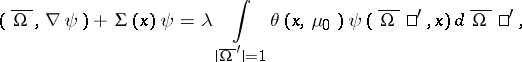(1)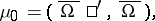with boundary condition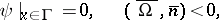(2)

whereis the boundary of a convex bounded domain. If the scatter indicatrixis an even function of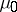, transition to the new unknown functionreduces the problem (1), (2) to self-adjoint form. In the problem thus obtained, Vladimirov's variational principle for the smallest eigenvalue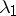states thatis the minimum of the functional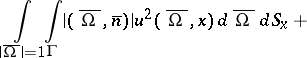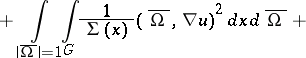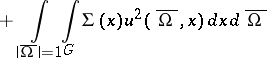on the set of functionswhich satisfy the conditionThe corresponding (non-negative) eigenfunction realizes the minimum of the functional . In this variational principle the respective boundary conditions are natural. Variational principles for higher eigenvalues and for the non-homogeneous problem are formulated in a similar manner.

The principle was first obtained by V.S. Vladimirov , and it yielded the optimum boundary conditions in the method of spherical harmonics (cf. Spherical harmonics, method of). Vladimirov's variational principle, in conjunction with finite difference methods, is extensively employed in numerical computations of neutron physics.